# Soma Cube

Solving Piet Hein’s Soma cube with Python.
programming
python
matplotlib
puzzles
Author

Aswin van Woudenberg

Published

October 20, 2023

The Soma cube is the brainchild of Danish mathematician, inventor, designer, writer and poet Piet Hein. The puzzle consists of seven pieces and the goal is to assemble these pieces into a 3x3x3 cube or other shapes.

Piet Hein came up with this puzzle during a lecture on quantum physics by Werner Heisenberg. Instead of paying attention to the renowned theoretical physicist, Piet prefered idling away his time by thinking up frivolous brainteasers. Such a waste, not to mention rude! What’s even worse is that people seemed to actually enjoy his puzzle. This meant that Piet Hein’s invention wasn’t just a time-waster for him but for everyone who got hooked on it.

Initially, the Soma cube was mostly known in Scandinavian countries, but things took a dark turn when Martin Gardner featured it in his Mathematical Games column in Scientific American in September 1958. Suddenly, the whole world got introduced to this time-waster.

As a self-proclaimed restorer of productivity, I hate to see people trifle away their lives trying to solve these inane puzzles. For this reason I’ve created a Soma solver in Python so people can go back to spending time on more fruitful pursuits.

Read on to learn how I did this.

## The Soma puzzle pieces

Each of the seven pieces has a different shape. I’ve given each shape a different color to ease visualizing solutions later on.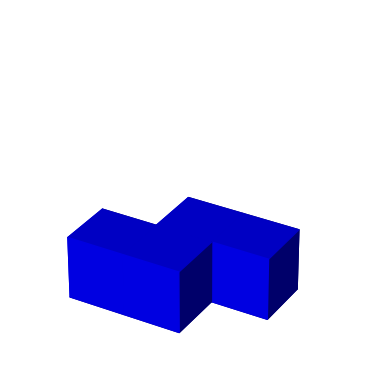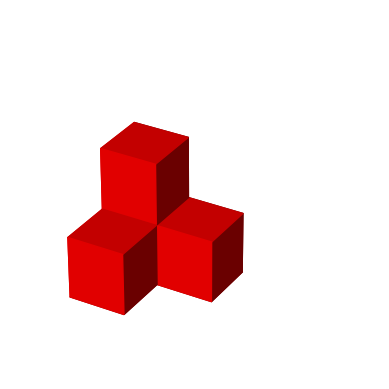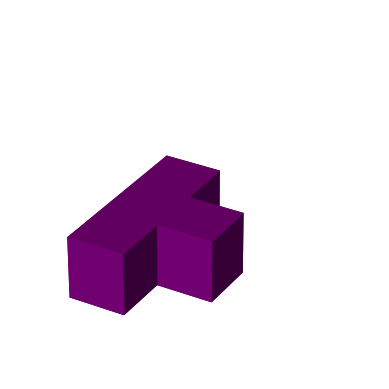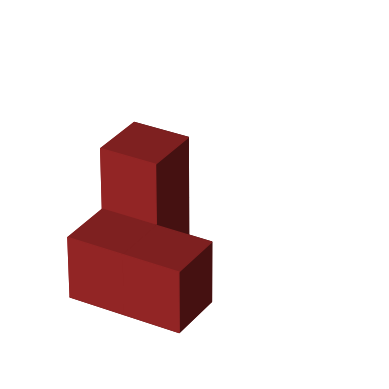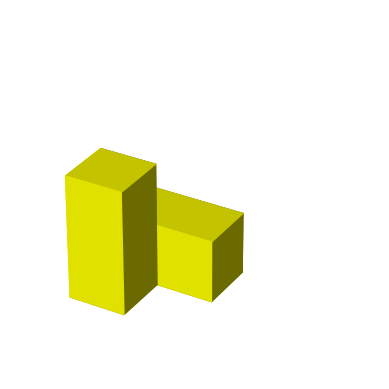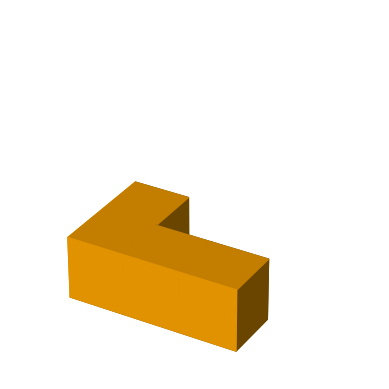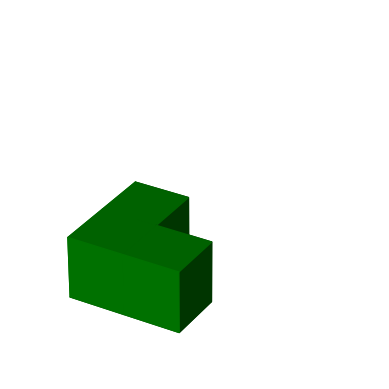As mentioned above, the objective is to assemble these pieces into various shapes, like a cube.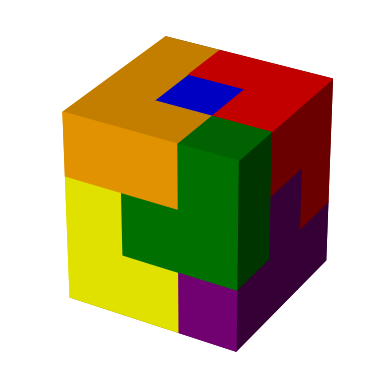## Importing libraries

Let’s start by importing some libraries. We’ll use `matplotlib` to visualize solutions in 3D.

``````import numpy as np
import matplotlib.pyplot as plt
import matplotlib.animation as animation
import ipywidgets as widgets

from IPython.display import HTML
from math import ceil``````

## Representing the pieces

We’ll represent each piece as a list of coordinates in 3D space. Each tuple is an (x, y, z) coordinate.

``````z = [(0,0,0),(1,0,0),(1,1,0),(2,1,0)] # blue piece
p = [(0,0,0),(0,1,0),(0,1,1),(1,1,0)] # red piece
t = [(0,0,0),(0,1,0),(1,1,0),(0,2,0)] # purple piece
b = [(0,0,0),(1,0,0),(0,1,0),(0,1,1)] # brown piece
a = [(0,0,0),(0,0,1),(0,1,0),(1,1,0)] # yellow piece
l = [(0,0,0),(1,0,0),(2,0,0),(0,1,0)] # orange piece
v = [(0,0,0),(1,0,0),(0,1,0)] # green piece``````

The letters `z`, `t`, `p`, `b`, `a`, `l`, and `v` look (with some imagination) like the pieces.

Let’s put all pieces and colors in a list so we can access them by index.

``````pieces = [z, p, t, b, a, l, v]
colors = ["blue", "red", "purple", "brown", "yellow", "orange", "green"]``````

## Visualizing the pieces and solution

Before writing the solver, let’s consider how we can create a 3D visualization of the Soma cube. I’ve chosen to use Matplotlib for its 3D rendering capabilities.

Matplotlib can draw voxels (3D pixels) which we can use to visualize a single piece, multiple pieces, or even the full solution in 3D. The following function takes a 3D numpy array that represents the voxels and plots them.

``````def plot_solution(colors, ax=None):
if not ax:
fig = plt.figure()

# axis with 3D projection

ax.set_aspect('equal')
ax.set_axis_off()

# draw each voxel with a color (each voxel unequal to None)
voxels = (colors != None)

ax.voxels(voxels, facecolors=colors, edgecolors=colors)``````

To use this function we need to call it with a 3D numpy array that represents each voxel.

``voxels = np.empty((3,3,3), dtype='object')``

Each element in this array is initialized to `None`.

``voxels``
``````array([[[None, None, None],
[None, None, None],
[None, None, None]],

[[None, None, None],
[None, None, None],
[None, None, None]],

[[None, None, None],
[None, None, None],
[None, None, None]]], dtype=object)``````

Let’s set each element of `voxels` to a color and call `plot_solution`. We can set elements seperately, like this:

``````voxels = 'yellow'
voxels = 'yellow'
voxels = 'yellow'
voxels = 'yellow'``````

Or we can set all elements at once.

``````voxels = np.array([
[['yellow', 'yellow', 'orange'],
['brown',  'yellow', 'orange'],
['brown',  'brown',  'orange']],
[['yellow', 'green',  'orange'],
['brown',  'blue',   'blue'],
['blue',   'blue',   'red']],
[['purple', 'green',  'green'],
['purple', 'purple', 'red'],
['purple', 'red',    'red']]
])``````
``````plot_solution(voxels)
plt.show()``````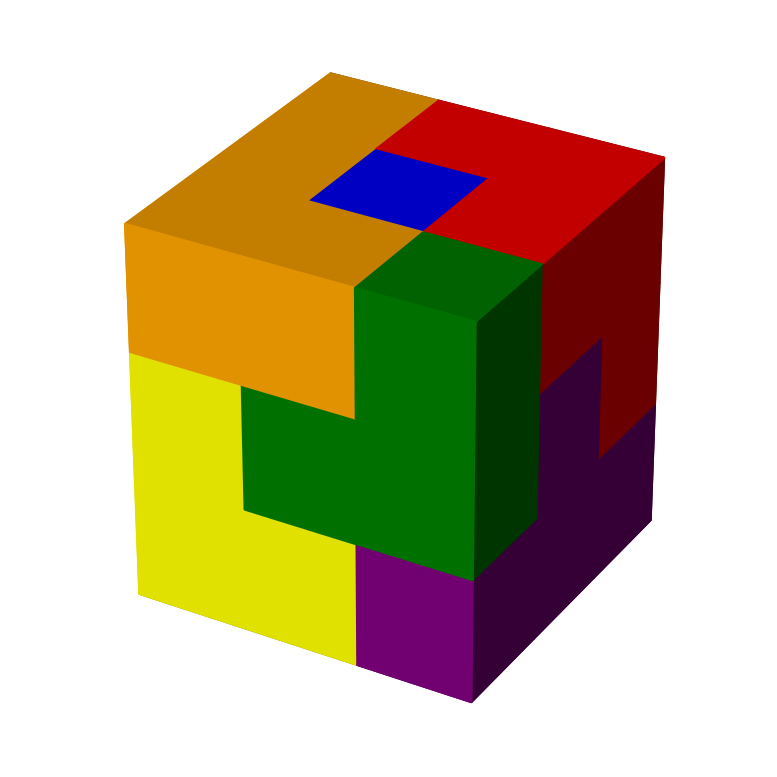This is the same solution as shown above and one of the 240 possible unique ways of packing the seven Soma pieces into a 3x3x3 cube.

Let’s see how we can visualize a single piece.

``````def plot_piece(piece, color, ax=None):
max_dim = np.max([np.max(piece) + 1, 3])
voxels = np.empty((max_dim, max_dim, max_dim), dtype='object')
for x, y, z in piece:
voxels[x][y][z] = color
plot_solution(voxels, ax)``````
``````plot_piece(z, "blue")
plt.show()``````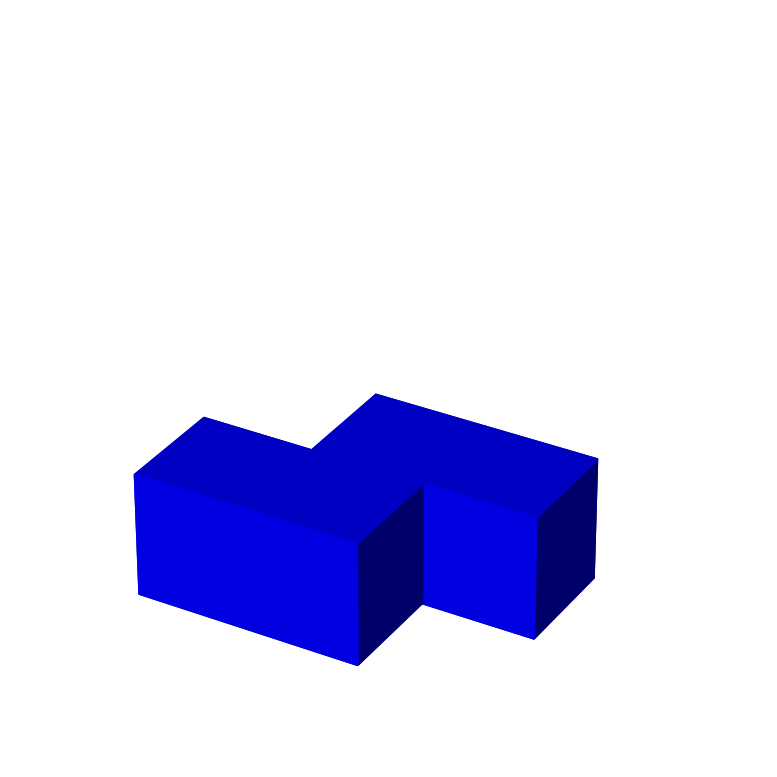## Animating a solution

In the solution above it was impossible to tell how some pieces were positioned. The following function animates the assembly of a solution by adding pieces one by one.

``````def animate_solution(colors):
w, d, h = colors.shape

show_order = []
for a in range(1,max(w, d, h)+1):
for z in range(min(a,h)):
for x in range(min(a,w)):
for y in range(d-1,d-1-min(a,d),-1):
color = colors[x][y][z]
if not (color is None or color in show_order):
show_order.append(color)

fig = plt.figure()

def update(frame):
ax.clear()
ax.set_aspect('equal')
ax.set_axis_off()

voxels = np.in1d(colors, show_order[:frame+1]).reshape(colors.shape)

ax.voxels(voxels, facecolors=colors, edgecolors=colors)

plt.close()

anim = animation.FuncAnimation(fig, update, frames=len(show_order), interval=1000)

return anim``````
``````anim = animate_solution(voxels)
HTML(anim.to_jshtml(default_mode='once'))``````

Now it’s much clearer how a solution is constructed.

## Rotating pieces

We will implement the solver using a simple recursive backtracking algorithm. The solver will try to fit the pieces in their various orientations. For this reason, we define some helper functions to rotate a piece around an axis. I also defined an `identity` function that just returns the input as is. Its use will become clear later.

``````rotate_x = lambda cubelets: [(x, z, -y) for (x, y, z) in cubelets]
rotate_y = lambda cubelets: [(z, y, -x) for (x, y, z) in cubelets]
rotate_z = lambda cubelets: [(-y, x, z) for (x, y, z) in cubelets]
identity = lambda cubelets: cubelets``````

For instance, to rotate piece `z` around the x-axis we can make use of `rotate_x`.

``rotate_x(z)``
``[(0, 0, 0), (1, 0, 0), (1, 0, -1), (2, 0, -1)]``

We can see that rotating a piece may make some coordinates negative. The following function translates a piece so that all `x`, `y`, `z` coordinates are minimal but positive.

``````def translate(piece):
d_x, d_y, d_z = np.min(np.array(piece), axis=0) * -1
return [(x + d_x, y + d_y, z + d_z) for (x, y, z) in piece]``````

Now we can do the same rotation as above but end up with coordinates that are all positive.

``translate(rotate_x(z))``
``[(0, 0, 1), (1, 0, 1), (1, 0, 0), (2, 0, 0)]``

We will use this `translate` function when we display individual pieces.

## Generating all orientations for each piece

Using the functions defined above we can generate all orientations for each piece. Several transformations are performed one after another. The `identity` function just returns the original orientation.

``````def generate_rotations(piece):
orientations = []
for f_a in [identity, rotate_x,  rotate_y, rotate_z]:
for f_b in [identity, rotate_x,  rotate_y, rotate_z]:
for f_c in [identity, rotate_x,  rotate_y, rotate_z]:
for f_d in [identity, rotate_x,  rotate_y, rotate_z]:
for f_e in [identity, rotate_x,  rotate_y, rotate_z]:
rot_piece = sorted(f_a(f_b(f_c(f_d(f_e(piece))))))
min_x, min_y, min_z = rot_piece
trans_rot_piece = [(x - min_x, y - min_y, z - min_z) for x, y, z in rot_piece]
if trans_rot_piece not in orientations:
orientations.append(trans_rot_piece)

return orientations``````

We can call this function for one piece.

``generate_rotations(z)``
``````[[(0, 0, 0), (1, 0, 0), (1, 1, 0), (2, 1, 0)],
[(0, 0, 0), (1, 0, -1), (1, 0, 0), (2, 0, -1)],
[(0, 0, 0), (0, 0, 1), (0, 1, -1), (0, 1, 0)],
[(0, 0, 0), (0, 1, 0), (1, -1, 0), (1, 0, 0)],
[(0, 0, 0), (1, -1, 0), (1, 0, 0), (2, -1, 0)],
[(0, 0, 0), (0, 1, 0), (0, 1, 1), (0, 2, 1)],
[(0, 0, 0), (0, 0, 1), (1, 0, 1), (1, 0, 2)],
[(0, 0, 0), (0, 1, -1), (0, 1, 0), (0, 2, -1)],
[(0, 0, 0), (1, 0, 0), (1, 0, 1), (2, 0, 1)],
[(0, 0, 0), (0, 1, 0), (1, 1, 0), (1, 2, 0)],
[(0, 0, 0), (0, 0, 1), (0, 1, 1), (0, 1, 2)],
[(0, 0, 0), (0, 0, 1), (1, 0, -1), (1, 0, 0)]]``````

Or we can apply this function to every piece in the `pieces` list we defined above.

``orientations = list(map(generate_rotations, pieces))``

We can display all orientations for each piece. Note how we apply `translate` to make sure all coordinates end up in the positive quadrant.

``````cols = 8
total_number_of_orientations = sum(list(map(len,orientations)))
rows = ceil(total_number_of_orientations / cols)
fig, axs = plt.subplots(rows, cols, figsize=(cols*3,rows*3),
subplot_kw= { 'projection':'3d' }, gridspec_kw = {'wspace':0.0, 'hspace':0.0})

fig.suptitle(f"Orientations", fontsize=16)

count = 0
for i in range(len(pieces)):
for j in range(len(orientations[i])):
plot_piece(translate(orientations[i][j]), colors[i], axs[count // cols, count % cols])
count += 1

while count < cols * rows:
axs[count // cols, count % cols].set_axis_off()
count += 1

plt.show()``````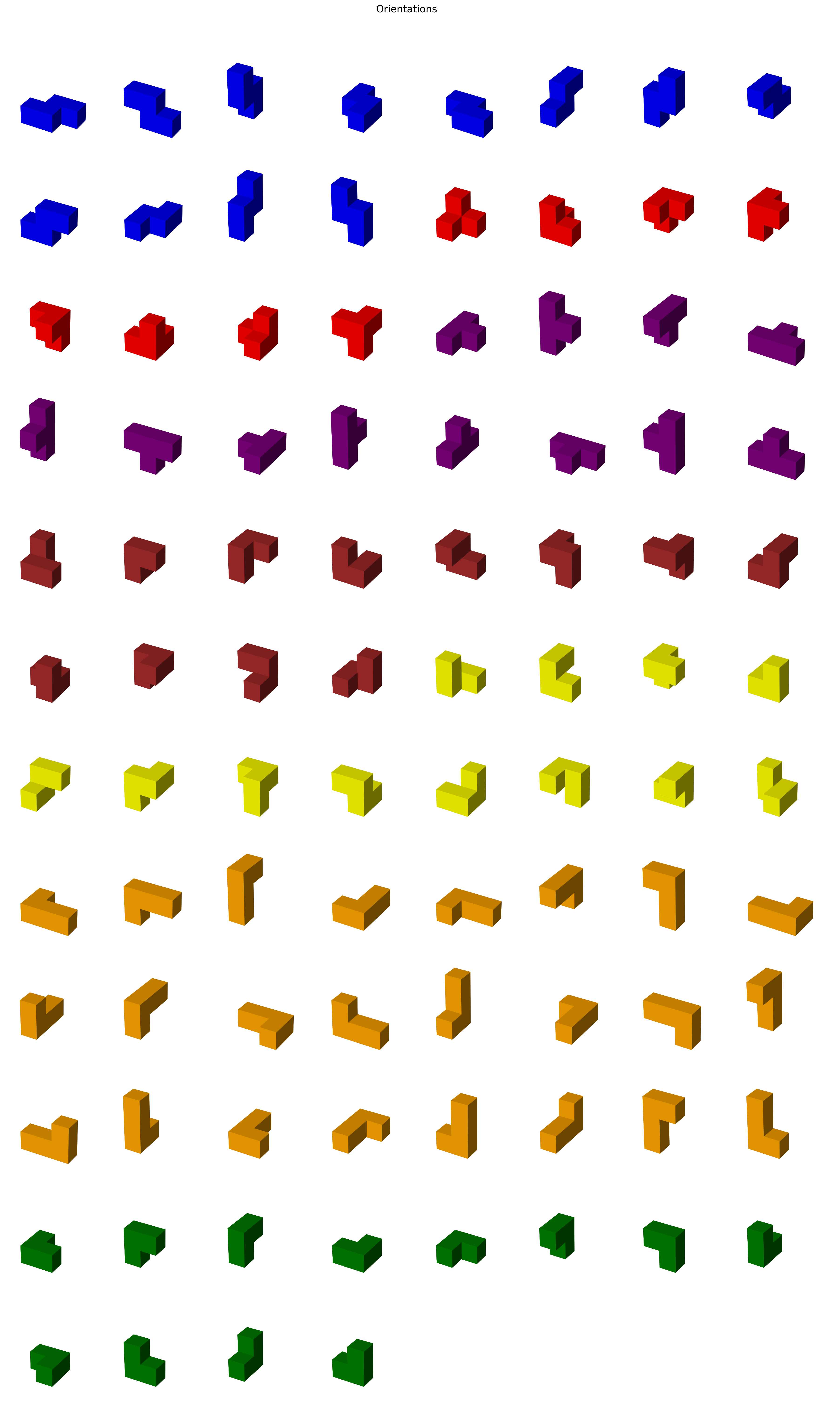In the solver below, for each piece we’ll see which orientation fits where.

## Writing the solver

We’re finally ready to work on the solver for the Soma cube. The solver will take in a list of coordinates (that make up the cube that we want to fill up with pieces) and recursively search for a solution.

We can generate this list of coordinates using a simple list comprehension.

``cube_coordinates = [(x, y, z) for x in range(3) for y in range(3) for z in range(3)]``
``cube_coordinates``
``````[(0, 0, 0),
(0, 0, 1),
(0, 0, 2),
(0, 1, 0),
(0, 1, 1),
(0, 1, 2),
(0, 2, 0),
(0, 2, 1),
(0, 2, 2),
(1, 0, 0),
(1, 0, 1),
(1, 0, 2),
(1, 1, 0),
(1, 1, 1),
(1, 1, 2),
(1, 2, 0),
(1, 2, 1),
(1, 2, 2),
(2, 0, 0),
(2, 0, 1),
(2, 0, 2),
(2, 1, 0),
(2, 1, 1),
(2, 1, 2),
(2, 2, 0),
(2, 2, 1),
(2, 2, 2)]``````

The solver will yield the same list but each tuple will have an extra element indicating the color that coordinate will get after all pieces have been placed.

We’ll use a list of booleans to keep track of which pieces have been placed.

``pieces_used = [False] * 7``
``````def solve_soma_(solution, i):
if i == 27:
yield solution
else:
x, y, z, _ = solution[i]
for piece in range(7):
if not pieces_used[piece]:
for orientation in orientations[piece]:
empty_coords = [(x + d_x, y + d_y, z + d_z, None) for (d_x, d_y, d_z) in orientation]
if all([tup in solution for tup in empty_coords]):
# placing piece: replace None with color
pieces_used[piece] = True
filled_coords = [(x + d_x, y + d_y, z + d_z, colors[piece]) for (d_x, d_y, d_z) in orientation]
new_solution = sorted([tup for tup in solution if tup not in empty_coords] + filled_coords)

# find next empty coordinate
j = i
while j < 27 and new_solution[j]:
j += 1

# continue search
yield from solve_soma_(new_solution, j)
pieces_used[piece] = False``````
``````def solve_soma(coordinates):
global pieces_used
pieces_used = [False] * 7

solution = sorted([(x, y, z, None) for x, y, z in coordinates])
yield from solve_soma_(solution, 0)``````

You call the `solve_soma` function with a list of coordinates representing the space that will be packed with Soma pieces. The actual algorithm is implemented in `solve_soma_`. The algorithm does the following:

1. It checks if all coordinates are filled. If so, it yields the solution.
2. For each possible orientation of a piece, it checks if it can be placed from the current coordinate without overlapping with filled coordinates that are already in the solution.
3. If a valid placement is found, it recursively explores how to fill from the next empty coordinate.
4. After exploring a piece and its orientation, it tries other possibilities.

Different solutions may be duplicates of each other in the sense that one solution is a rotation of another.

## Finding and displaying a solution

The solver is a generator function that yields all solutions one by one. Let’s start by just displaying the first solution it finds.

The following function finds the first solution given a list of coordinates to fill and plots this solution.

``````def solve_and_plot_first_solution(coordinates, ax=None):
# instantiate generator
gen = solve_soma(coordinates)

# find first solution
solution = next(gen)

# find voxel space size
max_dim = np.max(coordinates) + 1

# fill 3D voxel array and plot it
voxels = np.empty((max_dim, max_dim, max_dim), dtype='object')
for x, y, z, color in solution:
voxels[x][y][z] = color

plot_solution(voxels, ax)``````
``````solve_and_plot_first_solution(cube_coordinates)
plt.show()``````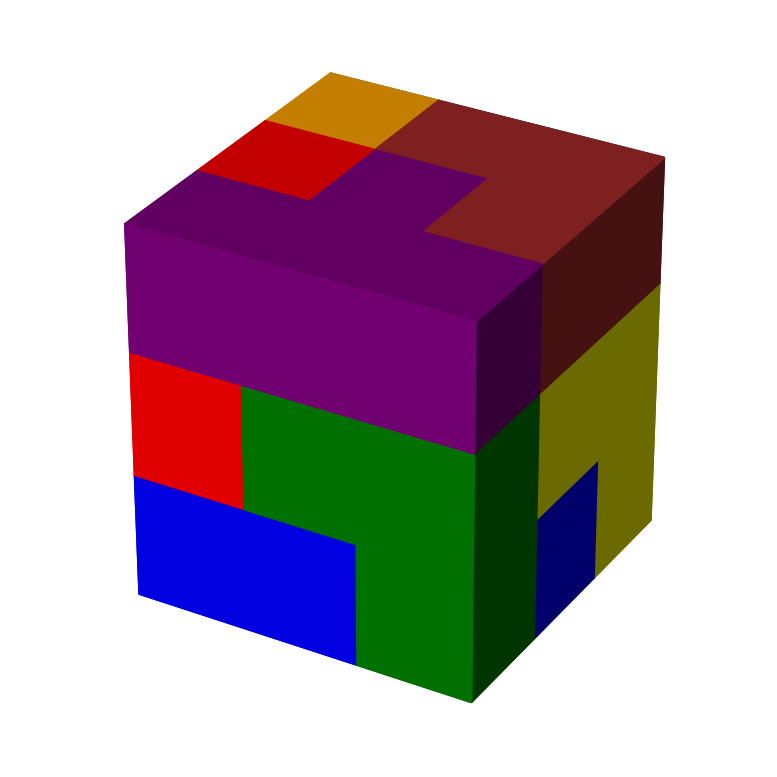We’ll also define a function that finds the first solution and then animates it.

``````def solve_and_animate_first_solution(coordinates):
# instantiate generator
gen = solve_soma(coordinates)

# find first solution
solution = next(gen)

# find voxel space size
max_dim = np.max(coordinates) + 1

# fill 3D voxel array and plot it
voxels = np.empty((max_dim, max_dim, max_dim), dtype='object')
for x, y, z, color in solution:
voxels[x][y][z] = color

anim = animate_solution(voxels)
display(HTML(anim.to_jshtml(default_mode='once')))``````
``solve_and_animate_first_solution(cube_coordinates)``

## Creating other figures

We called the solver with `cube_coordinates` that contains the coordinates that make up a cube. Instead of a cube, we can also pass a sorted list of coordinates that make up another shape.

Let’s look at some examples.

``````pyramid_coordinates = sorted(
[(x, y, 0) for x in range(1, 4) for y in range(5)] +
[(x, y, 0) for x in [0, 4] for y in range(1, 4)] +
[(2, y, 1) for y in range(1, 4)] +
[(1, 2, 1), (3, 2, 1), (2, 2, 2)]
)

turtle_coordinates = sorted(
[(x, y, z) for x in range(1, 4) for y in range(1, 4) for z in range(2)] +
[(4, 2, z) for z in range(3)] +
[(0, 2, 0), (1, 0, 0), (1, 4, 0), (3, 0, 0), (3, 4, 0), (5, 2, 2)]
)

tower_coordinates = sorted(
[(x, y, z) for x in range(2) for y in range(2) for z in range(7) if (x, y, z) != (1, 0, 6)]
)

bear_coordinates = sorted(
[(x, y, 0) for x in range(3) for y in range(2)] +
[(x, 1, z) for x in range(3) for z in range(1, 6)] +
[(x, 0, z) for x in [0, 2] for z in [1, 3, 4]]
)

tunnel_coordinates = sorted(
[(x, y, 0) for x in range(5) for y in range(3) if x != 2] +
[(x, y, 1) for x in range(1, 4) for y in range(3) if x != 2] +
[(x, y, 2) for x in range(1, 4) for y in range(3)]
)

tub_coordinates = sorted(
[(x, y, 0) for x in range(5) for y in range(3)] +
[(x, y, 1) for x in range(5) for y in range(3) if x in [0, 4] or y in [0, 2]]
)

airplane_coordinates = sorted(
[(x, y, 0) for x in range(1, 6) for y in range(7) if x == 3 or y in [0, 1]] +
[(x, y, 1) for x in range(7) for y in range(7) if y == 0 or (x == 3 and y != 5)]
)

bench_coordinates = sorted(
[(x, y, z) for x in range(5) for y in range(2) for z in range(3) if y == 1 or x in [0, 4]] +
[(x, 0, 1) for x in range(1, 4)] +
[(x, 1, 3) for x in range(1, 4)]
)

duck_coordinates = sorted(
[(x, y, z) for x in range(4) for y in range(3) for z in range(2)] +
[(3, 1, 2), (3, 1, 3), (4, 1, 3)]
)

[(x, y, z) for x in range(3) for y in range(3) for z in range(5) if (2-x) + y >= z]
)

chair_coordinates = sorted(
[(x, y, z) for x in range(3) for y in range(3) for z in range(2)] +
[(x, 2, z) for x in range(3) for z in range(2, 5)]
)

castle_coordinates = sorted(
[(x, y, 0) for x in range(5) for y in range(5) if (x, y) not in [(0,2), (4,2)]] +
[(0, 0, 1), (0, 4, 1), (4, 0, 1), (4, 4, 1)]
)

dog_coordinates = sorted(
[(x, y, 0) for x in range(6) for y in range(3) if (x, y) not in [(3, 0), (3, 2), (5, 1)]] +
[(x, y, 1) for x in range(5) for y in range(3) if x == 4 or y == 1] +
[(x, 1, z) for x in range(3, 6) for z in range(2, 4) if (x, z) != (5, 3)]
)

cross_coordinates = sorted(
[(x, y, z) for x in range(3) for y in range(3) for z in range(2)] +
[(1, y, 2) for y in range(3)] +
[(1, 1, z) for z in range(3, 7)] +
[(0, 1, 5), (2, 1, 5)]
)

elephant_coordinates = sorted(
[(0, 0, 0), (3, 0, 0), (0, 2, 0), (3, 2, 0)] +
[(x, y, 1) for x in range(4) for y in range(3) if (x, y) != (3, 1)] +
[(x, 1, z) for x in range(5) for z in range(2, 4) if (x, z) != (0, 3)] +
[(4, 1, 1), (2, 0, 2), (2, 2, 2)]
)

stairs_coordinates = sorted(
[(x, y, z) for y in range(3) for z in range(3) for x in range(4-z)]
)

snake_coordinates = sorted(
[(x, y, z) for y in range(4) for z in range(2) for x in range(2*(3-y), (2*(3-y))+3)] +
[(x, 0, z) for x in range(8, 10) for z in range(2, 4) if x == 8 or z == 3]
)

skyscraper_coordinates = sorted(
[(x, y, 0) for x in range(3) for y in range(3)] +
[(x, y, z) for x in range(2) for y in range(1, 3) for z in range(1, 5)] +
[(1, 1, 5), (1, 1, 6)]
)

wall_coordinates = sorted(
[(x, x - 1, 0) for x in range(1, 6)] +
[(x, x, z) for x in range(6) for z in range(2)] +
[(x, x + 1, z) for x in range(5) for z in range(2)]
)``````

Let’s place these figures and their names into lists.

``````figure_names = ["Cube", "Pyramid", "Turtle", "Tower", "Bear", "Tunnel", "Tub", "Airplane",
"Bench", "Duck", "Cascade", "Chair", "Castle", "Dog", "Cross", "Elephant",
"Stairs", "Snake", "Skyscraper", "Wall"
]
figure_coordinates = [
cube_coordinates, pyramid_coordinates, turtle_coordinates,
tower_coordinates, bear_coordinates, tunnel_coordinates,
tub_coordinates, airplane_coordinates, bench_coordinates,
castle_coordinates, dog_coordinates, cross_coordinates,
elephant_coordinates, stairs_coordinates, snake_coordinates,
skyscraper_coordinates, wall_coordinates
]``````

You can add more figures if you want. Just make sure that each list contains exactly 27 coordinates.

We can plot all figures as subplots.

``````cols = 5
number_of_figures = len(figure_coordinates)
rows = ceil(number_of_figures / cols)
fig, axs = plt.subplots(rows, cols, figsize=(cols*3,rows*3),
subplot_kw= { 'projection':'3d' }, gridspec_kw = {'wspace':0.5, 'hspace':0.0})

fig.suptitle(f"Figures", fontsize=16)

count = 0
for i in range(number_of_figures):
solve_and_plot_first_solution(figure_coordinates[i], axs[count // cols, count % cols])
axs[count // cols, count % cols].set_title(figure_names[i])
count += 1

while count < cols * rows:
axs[count // cols, count % cols].set_axis_off()
count += 1

plt.show()``````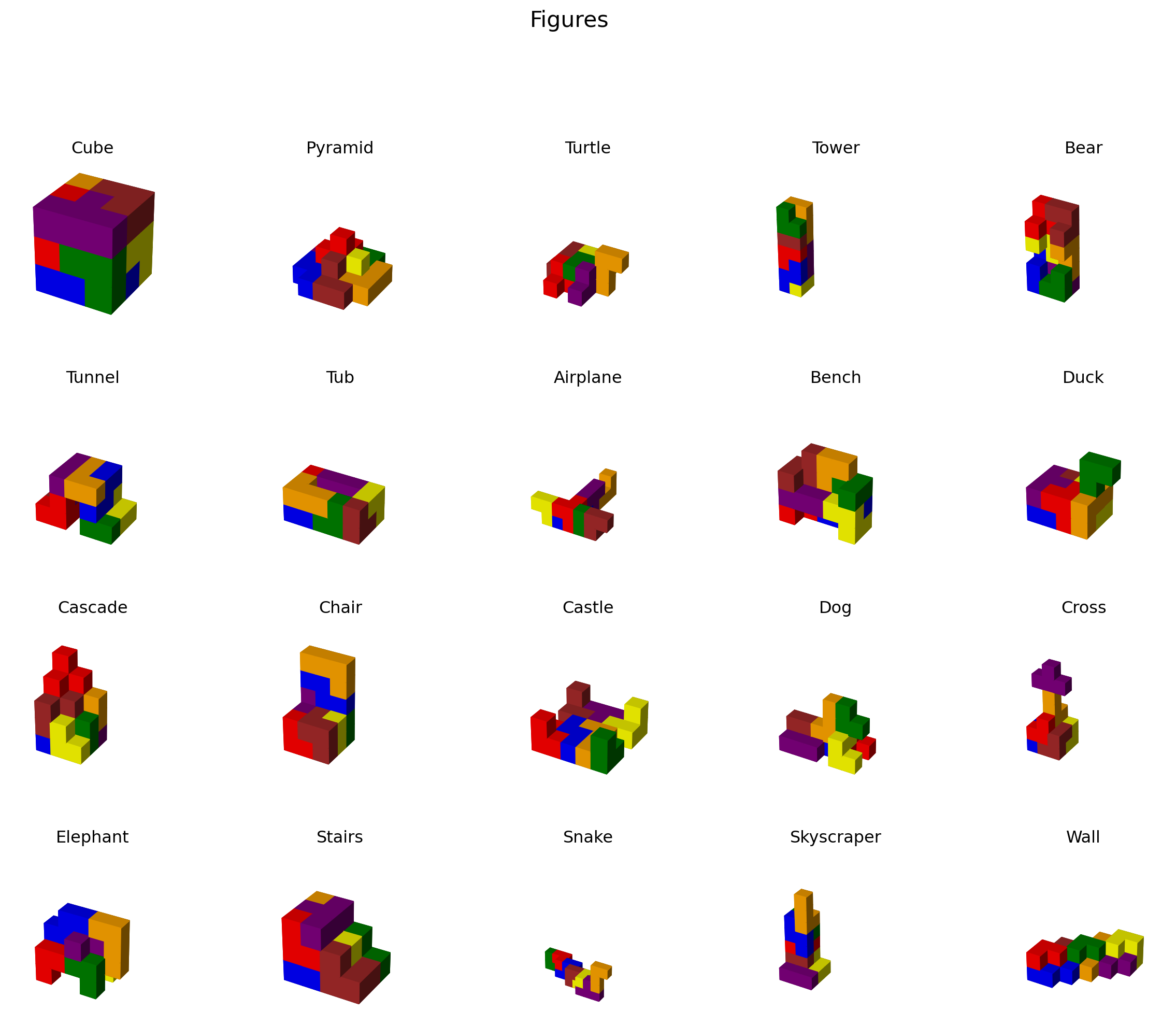I have to admit that some figures are pretty cute.

## Showing solutions one by one

Most figures can be assembled in more than one way. Using some widgets we can put together a simple GUI that lets us select a figure, solve it and iterate over the solutions.

We’ll use a dropdown menu, a button, an output widget, and a label widget. The solutions are shown as an animation we can step through.

``````figure_dropdown = widgets.Dropdown(options=zip(figure_names, figure_coordinates), value=cube_coordinates, description="Figure: ")
next_button = widgets.Button(description="Next")
hbox = widgets.HBox([figure_dropdown, next_button])
output = widgets.Output()
label = widgets.Label()

display(hbox, output, label)``````

To keep track of which solution to show next, we declare the following global variables:

``````gen = None
solution = None``````

The gen is a generator object that generates solutions for a given figure using the `solve_soma` function. The solution variable stores the next solution to be displayed. It is updated each time the “Next” button is clicked to display the next solution.

``````def figure_dropdown_change(change):
global gen
global solution

if change['type'] == 'change' and change['name'] == 'value':
value = change['new']
next_button.layout.display = 'none'

output.clear_output()

gen = solve_soma(value)
try:
solution = next(gen)
with output:
# find voxel space size
max_dim = np.max(value) + 1

# fill 3D voxel array and plot it
voxels = np.empty((max_dim, max_dim, max_dim), dtype='object')
for x, y, z, color in solution:
voxels[x][y][z] = color

anim = animate_solution(voxels)
display(HTML(anim.to_jshtml(default_mode='once')))
except StopIteration:
label.value = "No solutions found."
return

try:
solution = next(gen).copy()
label.value = ""
next_button.layout.display = 'inline-block'
except StopIteration:
label.value = ""

figure_dropdown.observe(figure_dropdown_change)``````

The `figure_dropdown_change` function handles changes to the figure dropdown menu. It clears the output widget, displays the first solution, and stores the next solution in the solution variable (if any).

``````def on_next_button_clicked(b):
global gen
global solution

output.clear_output(wait=True)
with output:
# find voxel space size
max_dim = np.max(figure_dropdown.value) + 1

# fill 3D voxel array and plot it
voxels = np.empty((max_dim, max_dim, max_dim), dtype='object')
for x, y, z, color in solution:
voxels[x][y][z] = color

anim = animate_solution(voxels)
display(HTML(anim.to_jshtml(default_mode='once')))

try:
solution = next(gen)
except StopIteration:
label.value = ""
next_button.layout.display = 'none'

next_button.on_click(on_next_button_clicked)

figure_dropdown_change({'type': 'change', 'name': 'value', 'new': figure_dropdown.value})``````

The `on_next_button_clicked` function handles clicks on the “Next” button by clearing the output widget, displaying the current solution, and generating the next solution.

## Solving your own Soma puzzles

There you have it! No more wasting time on solving Soma puzzles. If you want to experiment with an interactive version of the solver, you can check out on of the following links:

Now go do something useful!